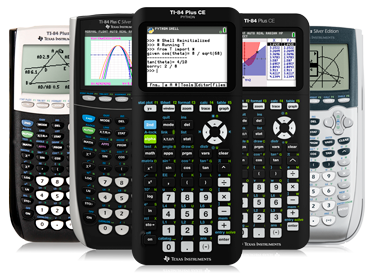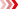Algebra II
Find activities that support your lesson plans

Challenge students to use and think about how technology can be used to model, analyze and explain math with standards-aligned activities in eight algebra II topics.

Activities can be used as is or edited to support specific objectives, align with popular text books and include technology tips to help you focus the learning and address student misconceptions.# Featured Activities

## Arithmetic Sequences & Series

Students use formulas to find the differences of the consecutive terms, plot a scatter plot of each sequence, and determine that sequences with common differences (called arithmetic sequences) have scatter plots whose points form a straight line.## Ain’t No River Wide Enough

Students simulate the process a surveyor would use to measure the width of a river by measuring length on one side of the river and angles formed by various reference points.## Average Orange

Students explore the area under the normal curve between various x-values and use a model to find what percent of the area lies within 1, 2, and 3 standard deviations of the mean weights. Percentiles are defined and calculated.